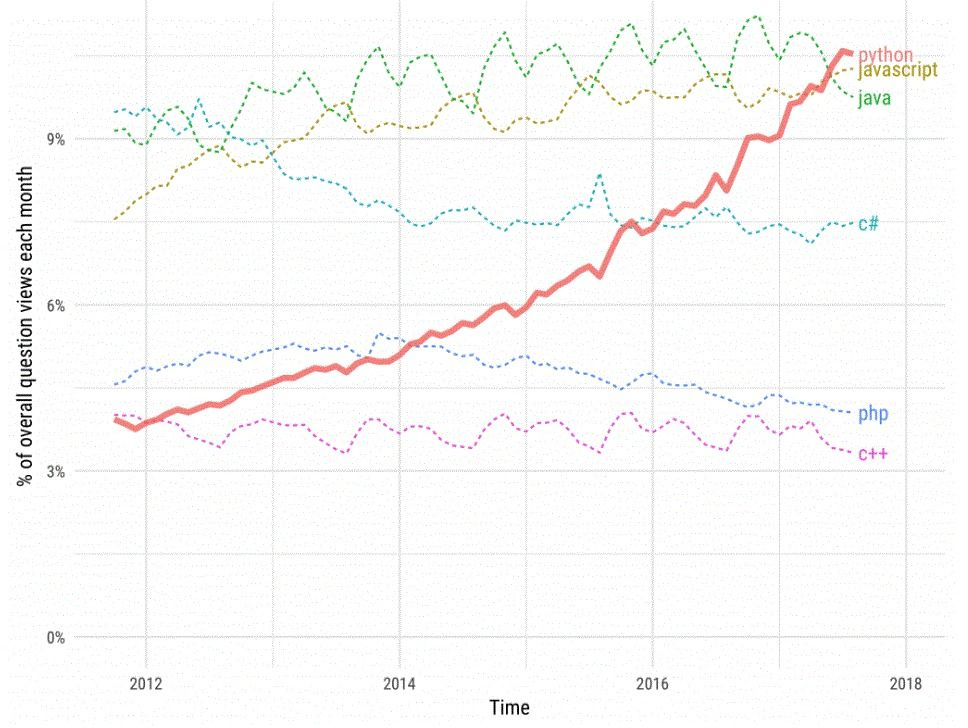# 22 Hottest Python Tricks for Efficient CodingIn the wake of the pandemic, Python coding skills have become especially in demand. To thrive in such a scenario, you need to know some tricks that can cut down your development hours and improve code runtime.

This article will walk you through 22 Python programming tricks for efficient coding.

## Why Python?

Right from development to maintenance, Python programming increases developers’ productivity in software development. According to a survey, it has quickly gained popularity among developers over the past decade.Img Source: Stack Overflow

Here are some Python code examples you need to implement in Python programming. Whether you have deployed just one or two projects, all these tricks will come in handy for future projects.

## Top Python tricks for efficient programming

To keep things simple and transparent, the tricks have been categorized based on a few key aspects such as lists, strings, matrix, dictionary, etc.

### Lists

Trick 1: Flatten the lists

import itertools
a = [[1, 2], [3, 4], [5, 6]]
b = list(itertools.chain.from_iterable(a))
print(b)

Output:
[1, 2, 3, 4, 5, 6]

Trick 2: Reverse a list

a=[“10”,”9",”8",”7"]
print(a[::-1])

Output:
10
9
8
7

Trick 3: Combining different lists

a=[‘a’,’b’,’c’,’d’]
b=[‘e’,’f’,’g’,’h’]
for x, y in zip(a, b):
print(x,y)

Output:
a e
b f
c g
d h

Trick 4: Negative indexing lists

a = [0, 1, 2, 3, 4, 5, 6, 7, 8, 9, 10]
a[-3:-1]

Output:
[8, 9]

Trick 5: Analyzing the most frequent on the list

a = [1, 2, 3, 4, 2, 2, 3, 1, 4, 4, 4]
print(max(set(a), key = a.count))

Output:
4

### Strings

Trick 6: Reversing the string

a=”python”
print(“Reverse is”, a[::-1])

Output:
Reverse is nohtyp

Trick 7: Splitting the string

a="Python is the language of the future"
b=a.split()
print(b)

Output:
[‘Python’, ‘is’, ‘the’, ‘language’, ‘of’, 'the’, ‘future’]

Trick 8: Printing out multiple values of strings

print(“on”*3+’ ‘+”off”*2)

Output:
ononon offoff

Trick 9: Creating a single string

a = [“I”, “am”, “not”, “available”]
print(“ “.join(a))

Output:
I am not available

Trick 10: Checking if two words are anagrams

from collections import Counter
def is_anagram(str1, str2):
return Counter(str1) == Counter(str2)
print(is_anagram(‘taste’, ‘state))
print(is_anagram(‘beach’, ‘peach’))

Output:
True
False

### Matrix

Trick 11: Transposing a matrix

mat = [[8, 9, 10], [11, 12, 13]]
new_mat=zip(*mat)
for row in new_mat:
print(row)

Output:
(8, 11)
(9, 12)
(10, 13)

### Operators

Trick 12: Chaining comparison operators

a = 17
b = 21
c = 11
print(c < a)
print(a < b)

Output:
True
True
True

### Dictionary

Trick 13: Inverting the Dictionary

dict1={‘a’: 1, ‘b’: 2,‘c’: 3,‘d’: 4,‘e’: 5,‘f’: 6, ‘g’: 7}
dict2={v: k for k, v in dict1.items()}
print(dict2)

Output:
{1: ‘a’, 2: ‘b’, 3: ‘c’, 4: ‘d’, 5: ‘e’, 6: ‘f’, 7: ‘g’}

Trick 14: Iterating value pairs and dictionary keys

dict1={‘a’: 1, ‘b’: 2, ‘c’: 3, ‘d’: 4, ‘e’: 5, ‘f’: 6}
for a, b in dict1.iteritems():
print (‘{: {}’.format(a,b))

Output:
a: 1
b: 2
c: 3
d: 4
f: 6

Trick 15: Merging multiple dictionaries

x = {'a': 1, 'b': 2}
y = {'b': 3, 'c': 4}
z = {**x, **y}
print(z)

Output:
{‘a’: 1, ‘b’: 3, ‘c’: 4}

### Initialization

Trick 16: Initializing empty spaces

a_list = list()
a_dict = dict()
a_map = map()
a_set = set()

Trick 17: Initializing lists filled with numbers

#listA contains 1000 1's
listA=*1000
#listB contains 1000 2's
listB=*1000

### Miscellaneous

Trick 18: Checking and analyzing the memory unit of an object

import sys
a=10
print(sys.getsizeof(a))

Output:
28

Trick 19: Swapping values

x, y = 13, 26
x, y = y, x
print(x, y)

Output:
26 13

### Map functions

Trick 20: Implementing the map function

In competitive coding, you might come across an input like this:
1234567890

To get the input as a list of numbers, perform the following:
list(map (int, input().split()))

Note: Always use the input() function irrespective of the type of input and convert it using the map function.

list(map(int, input("enter numbers:").split()))
enter numbers:1 2 3 4 5 6 7
[1, 2, 3, 4, 5, 6, 7]

The map function is one of the most useful in-built functions and features of Python.

### Collections module

Trick 21: Merging different lists

The Collections module allows you to remove duplicates from a list. In Java, you have to use the HashMap to remove duplicate modules, but it’s far easier in the case of Python.

print(list(set([1,2,3,4,3,4,5,6,7,8,9])))
[1, 2, 3, 4, 5, 6, 7, 8, 9]

You need to use extend() and append() in the lists while merging multiple lists.

a = [1, 2, 3, 4] # list 1
b = [ 5, 6, 7, 8, 9] # list 2

Note: >>> a.extend(b) will display one list.

a
[1, 2, 3, 4]

Note: >>> a.append(b) will display the list of list.

a
[1, 2, 3, 4 [5, 6, 7, 8, 9]]

### Language constructs

Trick 22: Writing code within functions

In Python, it is always better to write your code within functions.

def main():
for i in range(2**3):
print(x)
main()

The above code fragment is better than the one below:

for x in range(2**3):
print(x)

The CPython implementation saves time in the case of storing local variables.

Bonus tip

These useful Python tricks will help you code better and more efficiently. Here is a bonus tip that you should know and implement.

### Strings concatenation

str1 = ""
some_list = ["Welcome ", "To ", "Bonus ", “Tips ”]
print(str1.join(some_list))

Use the above code instead of:

str1 = ""
some_list = ["Welcome ", "To ", "Bonus ", “Tips ”]
for x in some_list:
str1 += x
print(str1)

Speed and, most of all, efficiency, are key to coding better. By incorporating the tips outlined in this article, you can significantly improve your Python programming skills. Give them a try in your next competitive coding event or other Python projects and notice the difference they make.### Press

What's up with Turing? Get the latest news about us here.### Blog